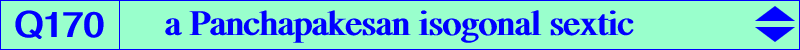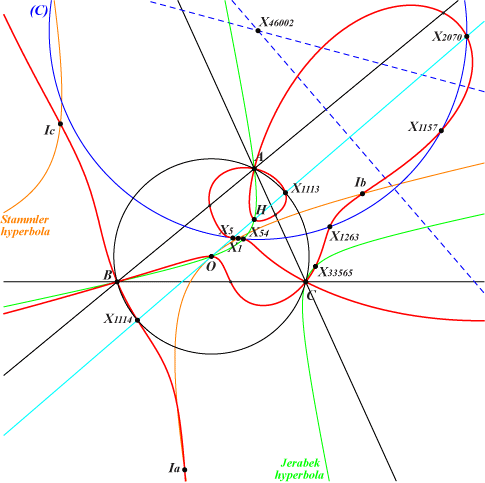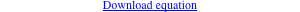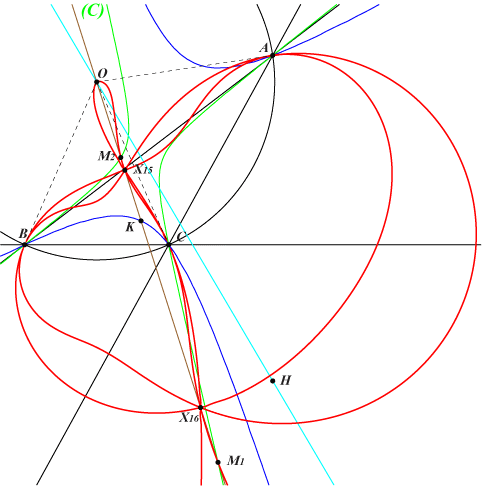too complicated to be written here. Click on the link to download a text file.X(1), X(3), X(4), X(5), X(54), X(1113), X(1114), X(1157), X(1263), X(2070), X(2574), X(2575), X(33565) excenters anti-points, see Table 77Panchapakesan circles are defined in the preamble just before X(46001) of ETC. Recall that the Panchapakesan circle PC(P) of P is orthogonal to the circumcircle (O) and always contains P, its isogonal conjugate gP, its inverse iP in (O) and igP. Now, if M is a given finite point not lying on (O), the locus of P such that PC(P) passes through M is generally a bicircular isogonal circum-sextic S(M) passing through M, gM, iM, giM and the six anti-points, see Q169. It also passes through igM and gigM (the antigonal of M) when M lies on Q038. S(M) meets the line at infinity again at the same points as the rectangular circum-hyperbola H(M) passing through gM, hence the isogonal transform of the line OM. Obviously, S(M) meets (O) at the isogonal conjugates of these infinite points and five double points, namely A, B, C, and the circular points at infinity. Note that S(M) and S(iM) are the same curve. When M = O, S(M) splits into the line at infinity, (O) and K003. Q021 = S(X5000) = S(X5001) and Q039 = S(X15) = S(X16) are two remarkable examples. Q170 is S(X5) or S(X2070). Its real asymptotes are parallel to those of the Jerabek and Stammler hyperbolas. They concur at X = X(30) x X(15108), on the lines {30,113), {140,389}. X is now X(46114) in ETC (2021-11-28). See also Q185 and Q186. *** A generalized property of Q021 and Q039 Q021 meets the sidelines of ABC again at the cevians of X(4) and the traces of the trilinear polar of X(6). Q039 meets the sidelines of ABC again at the cevians of X(1) and the traces of the trilinear polar of X(1). Now, if P is a point on K004, the sextic S(M) that passes through the cevians of P meets the sidelines of ABC again at the traces of the trilinear polar of Q = Psi(P), where Psi is the Cundy-Parry transformation defined in CL037.In this case, the points M and iM lie on a very complicated curve (Q) of degree 11 which is inversible in the circumcircle. (Q) meets the line at infinity at X(1154) and the circular points, each counted five times. A, B, C are double points with tangents passing through O. (Q) passes through X(5000), X(5001) on the Euler line, as seen for Q021. (Q) meets the Brocard axis at O, two quadruple points X(15) and X(16) as seen for Q039, and two remaining points M1, M2. M1, M2 are inverse in (O) and they also lie on the circum-conic passing through X(249), X(394). They are the base-points of a pencil of circles containing the circles {20,64,2071}, {22,858,1560}, {110,154,1636}, {112,2935,3184}, {115,2079,5504,15478,17838}. The corresponding sextic S(M1) = S(M2) is Q171.